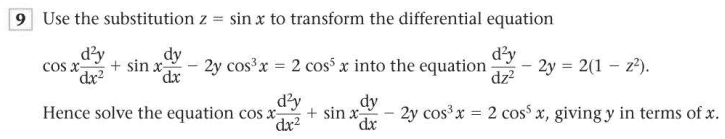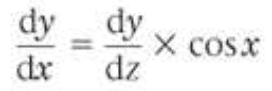# 2nd order differential equations

• trew

## Homework Statement## The Attempt at a Solution

I managed to find dy/dx as follows:But I'm having difficulty finding the second derivative. I've looked at examples using the chain rule but I'm still confused.

Would someone mind shedding some light on this for me?

#### Attachments

I managed to find dy/dx as follows:
This is not particular for dy/dx. It holds if you replace y by any function. In particular, what do you get if you replace y by dy/dx?

•trew

## Homework Equations

The product rule and the chain rule are relevant equations.

But I'm having difficulty finding the second derivative.

Part of applying the product rule to find ##\frac{d^2 y}{dx^2} = \frac{d}{dx} ( \frac{dy}{dz} cos(x))## requires evaluating the factor ##\frac{d}{dx} \frac{dy}{dz}##. The chain rule, in words, says "the derivative with respect to x of a function of z is equal to the derivative of the function with respect to z times the derivative of z with respect to x". As @Orodruin pointed out, you can apply this rule when "a function of z" is ##\frac{dy}{dz}##. What do you get when you differentiate ##\frac{dy}{dz}## with respect to ##z## ?

•trew